# WO2014108964A1 - Lighting apparatus, lamp using lighting apparatus, and vehicle lamp using lighting apparatus - Google Patents

Lighting apparatus, lamp using lighting apparatus, and vehicle lamp using lighting apparatus Download PDF

## Info

Publication number
WO2014108964A1
WO2014108964A1 PCT/JP2013/007429 JP2013007429W WO2014108964A1 WO 2014108964 A1 WO2014108964 A1 WO 2014108964A1 JP 2013007429 W JP2013007429 W JP 2013007429W WO 2014108964 A1 WO2014108964 A1 WO 2014108964A1
Authority
WO
WIPO (PCT)
Prior art keywords
frequency
value
switching
lighting
output current
Prior art date
Application number
PCT/JP2013/007429
Other languages
French (fr)
Japanese (ja)
Inventor

Original Assignee
パナソニック株式会社
Priority date (The priority date is an assumption and is not a legal conclusion. Google has not performed a legal analysis and makes no representation as to the accuracy of the date listed.)
Filing date
Publication date
Priority to JP2013-001929 priority Critical
Priority to JP2013001929A priority patent/JP6089273B2/en
Application filed by パナソニック株式会社 filed Critical パナソニック株式会社
Publication of WO2014108964A1 publication Critical patent/WO2014108964A1/en

• 238000006243 chemical reactions Methods 0.000 claims abstract description 38
• 239000003990 capacitor Substances 0.000 claims description 95
• 230000003247 decreasing Effects 0.000 claims description 10
• 230000001276 controlling effects Effects 0.000 claims description 3
• 238000010586 diagrams Methods 0.000 description 34
• 238000004804 winding Methods 0.000 description 19
• 238000000034 methods Methods 0.000 description 13
• 230000005540 biological transmission Effects 0.000 description 8
• 230000000875 corresponding Effects 0.000 description 8
• 230000001629 suppression Effects 0.000 description 8
• 210000004286 T(FH) Anatomy 0.000 description 7
• 230000001808 coupling Effects 0.000 description 7
• 238000010168 coupling process Methods 0.000 description 7
• 238000005859 coupling reactions Methods 0.000 description 7
• 230000001702 transmitter Effects 0.000 description 7
• 241000152160 Ira Species 0.000 description 5
• 230000000694 effects Effects 0.000 description 5
• 230000000630 rising Effects 0.000 description 5
• 238000004904 shortening Methods 0.000 description 5
• 239000007787 solids Substances 0.000 description 4
• 230000003287 optical Effects 0.000 description 3
• 101710051999 NRG2 Proteins 0.000 description 2
• 102100015657 Pro-neuregulin-2, membrane-bound isoform Human genes 0.000 description 2
• 238000009792 diffusion process Methods 0.000 description 2
• 238000009499 grossing Methods 0.000 description 2
• 238000005286 illumination Methods 0.000 description 2
• 230000001429 stepping Effects 0.000 description 2
• 230000003111 delayed Effects 0.000 description 1
• 238000009434 installation Methods 0.000 description 1
• 239000002184 metals Substances 0.000 description 1
• 230000003071 parasitic Effects 0.000 description 1
• 239000000758 substrates Substances 0.000 description 1

## Images

•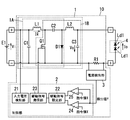•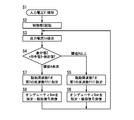•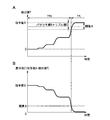•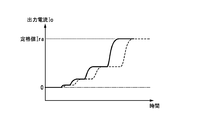••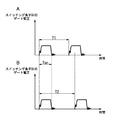•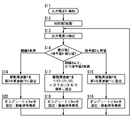•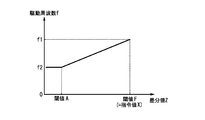•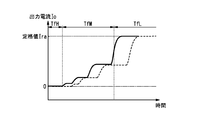••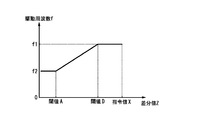•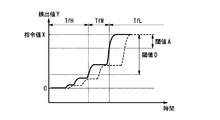•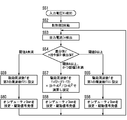•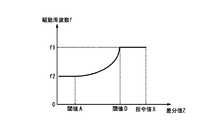•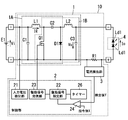•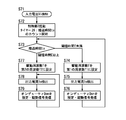•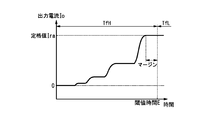•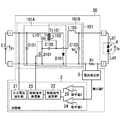•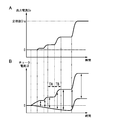•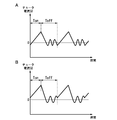•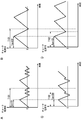•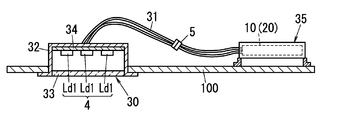•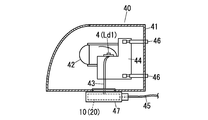•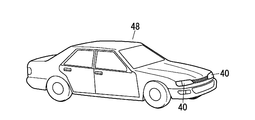## Classifications

• HELECTRICITY
• H02GENERATION; CONVERSION OR DISTRIBUTION OF ELECTRIC POWER
• H02MAPPARATUS FOR CONVERSION BETWEEN AC AND AC, BETWEEN AC AND DC, OR BETWEEN DC AND DC, AND FOR USE WITH MAINS OR SIMILAR POWER SUPPLY SYSTEMS; CONVERSION OF DC OR AC INPUT POWER INTO SURGE OUTPUT POWER; CONTROL OR REGULATION THEREOF
• H02M1/00Details of apparatus for conversion
• H02M1/36Means for starting or stopping converters
• HELECTRICITY
• H02GENERATION; CONVERSION OR DISTRIBUTION OF ELECTRIC POWER
• H02MAPPARATUS FOR CONVERSION BETWEEN AC AND AC, BETWEEN AC AND DC, OR BETWEEN DC AND DC, AND FOR USE WITH MAINS OR SIMILAR POWER SUPPLY SYSTEMS; CONVERSION OF DC OR AC INPUT POWER INTO SURGE OUTPUT POWER; CONTROL OR REGULATION THEREOF
• H02M3/00Conversion of dc power input into dc power output
• H02M3/005Conversion of dc power input into dc power output using Cuk converters
• HELECTRICITY
• H02GENERATION; CONVERSION OR DISTRIBUTION OF ELECTRIC POWER
• H02MAPPARATUS FOR CONVERSION BETWEEN AC AND AC, BETWEEN AC AND DC, OR BETWEEN DC AND DC, AND FOR USE WITH MAINS OR SIMILAR POWER SUPPLY SYSTEMS; CONVERSION OF DC OR AC INPUT POWER INTO SURGE OUTPUT POWER; CONTROL OR REGULATION THEREOF
• H02M3/00Conversion of dc power input into dc power output
• H02M3/02Conversion of dc power input into dc power output without intermediate conversion into ac
• H02M3/04Conversion of dc power input into dc power output without intermediate conversion into ac by static converters
• H02M3/10Conversion of dc power input into dc power output without intermediate conversion into ac by static converters using discharge tubes with control electrode or semiconductor devices with control electrode
• H02M3/145Conversion of dc power input into dc power output without intermediate conversion into ac by static converters using discharge tubes with control electrode or semiconductor devices with control electrode using devices of a triode or transistor type requiring continuous application of a control signal
• H02M3/155Conversion of dc power input into dc power output without intermediate conversion into ac by static converters using discharge tubes with control electrode or semiconductor devices with control electrode using devices of a triode or transistor type requiring continuous application of a control signal using semiconductor devices only
• H02M3/156Conversion of dc power input into dc power output without intermediate conversion into ac by static converters using discharge tubes with control electrode or semiconductor devices with control electrode using devices of a triode or transistor type requiring continuous application of a control signal using semiconductor devices only with automatic control of output voltage or current, e.g. switching regulators
• HELECTRICITY
• H05ELECTRIC TECHNIQUES NOT OTHERWISE PROVIDED FOR
• H05BELECTRIC HEATING; ELECTRIC LIGHTING NOT OTHERWISE PROVIDED FOR
• H05B45/00Circuit arrangements for operating light emitting diodes [LED]
• H05B45/10Controlling the intensity of the light
• HELECTRICITY
• H05ELECTRIC TECHNIQUES NOT OTHERWISE PROVIDED FOR
• H05BELECTRIC HEATING; ELECTRIC LIGHTING NOT OTHERWISE PROVIDED FOR
• H05B45/00Circuit arrangements for operating light emitting diodes [LED]
• H05B45/30Driver circuits
• H05B45/37Converter circuits
• BPERFORMING OPERATIONS; TRANSPORTING
• B60VEHICLES IN GENERAL
• B60QARRANGEMENT OF SIGNALLING OR LIGHTING DEVICES, THE MOUNTING OR SUPPORTING THEREOF OR CIRCUITS THEREFOR, FOR VEHICLES IN GENERAL
• B60Q1/00Arrangements or adaptations of optical signalling or lighting devices
• B60Q1/0088Details of electrical connections
• B60Q1/0094Arrangement of electronic circuits separated from the light source
• HELECTRICITY
• H02GENERATION; CONVERSION OR DISTRIBUTION OF ELECTRIC POWER
• H02MAPPARATUS FOR CONVERSION BETWEEN AC AND AC, BETWEEN AC AND DC, OR BETWEEN DC AND DC, AND FOR USE WITH MAINS OR SIMILAR POWER SUPPLY SYSTEMS; CONVERSION OF DC OR AC INPUT POWER INTO SURGE OUTPUT POWER; CONTROL OR REGULATION THEREOF
• H02M1/00Details of apparatus for conversion
• H02M1/14Arrangements for reducing ripples from dc input or output

## Abstract

A lighting apparatus (10) is provided with: a power conversion circuit (1), which is configured from a Cuk converter circuit that forms first and second converter circuits (1A, 1B), and which supplies an output current (Io) to a light source (4) when a switching element (Q1) is on/off driven; a current detecting unit (3) that detects the output current (Io); and a control unit (2), which performs feedback control such that the output current (Io) is at a target value by setting a duty ratio of the switching element (Q1) on the basis of a detection value (Y) of the output current (Io). The control unit (2) sets a drive frequency (f) of the switching element (Q1) higher for the startup time of the output current (Io) than a drive frequency (f) at which the output current (Io) is in a steady state.

## Description

Lighting device, lighting fixture using the same, and lighting fixture for vehicle

The present invention relates to a lighting device, a lighting fixture using the lighting device, and an in-vehicle lighting fixture.

In recent years, with the increase in efficiency and brightness of LEDs, the demand for LEDs as light sources for downlight illumination, straight tube illumination, and vehicle headlamps has increased. With the rapid spread of LEDs, there is an urgent need to develop a lighting device that lights the LEDs.

Also, in the in-vehicle industry, development of lighting devices for headlamps using LED light sources has been actively conducted, and in particular, cost reduction has been raised as an important issue. In order to reduce the cost of the lighting device, it is necessary to avoid the DC-DC converter method using an expensive large transformer and the control using a high bit microcomputer, and to adopt an inexpensive coil / small transformer or a low bit microcomputer. It can be said that it is one of the means.

Therefore, a Cuk converter circuit, which is composed of a choke coil without using a transformer and has stable input / output current ripple, has been attracting attention (see, for example, Japanese Patent Application Publication No. 2005-224094).

The Cuk converter circuit includes a first inductor, a second inductor, a capacitor (hereinafter, referred to as a coupling capacitor) connected between the first inductor and the second inductor, and a switching element. In the Cuk converter circuit, energy moves in the order of power source → first inductor → coupling capacitor → second inductor according to the switching operation of the switching element, and the energy stored in the second inductor is released to the outside.

The cost of the lighting device can be reduced by using the power conversion circuit composed of the above-described Cuk converter circuit and further adopting a control unit composed of an inexpensive low-bit microcomputer with a slow calculation speed.

However, when the above-described Cuk converter circuit is combined with a low-bit microcomputer having a low calculation speed, the following problem occurs.

In the case where the light source is rated-lit from the extinguished state, the control unit gradually increases the duty ratio of the switching element by feedback control, thereby increasing the output current of the power conversion circuit to the target value.

However, when the control unit is configured with a low-bit microcomputer with a slow calculation speed, feedback control of the output current (duty ratio update) becomes slow. Therefore, it is necessary to moderate the degree of increase in the duty ratio in order to suppress overshooting of the output current when the output current is raised to increase the output current from the zero state toward the target value.

However, in a period in which the duty ratio of the switching element is low, there is a possibility that a so-called reverse flow in which a current flows from the coupling capacitor toward the input side choke coil (first inductor) may occur. When backflow occurs, there is a possibility that a period during which the output current Io does not increase (hereinafter referred to as a stagnation period TA) may occur.

FIG. 19A shows an example of a waveform diagram of the output current Io of the Cuk converter circuit at startup. As shown in FIG. 19A, when the output current Io rises, the rising waveform of the output current Io is stepped by the stagnation period TA of the output current Io, and as a result, the output current Io becomes the rated value Ira (target Value) until it reaches (value) (the rise time of the output current Io) is delayed. Hereinafter, a period in which the output current Io increases is referred to as an increase period TB.

FIG. 19B shows a waveform diagram of the choke current Il flowing through the first inductor when the output current Io rises. Note that the solid line in FIG. 19B indicates the envelope of the peak value and the bottom value of the choke current Il that repeatedly increases and decreases. FIG. 20A shows an enlarged waveform diagram of the choke current Il during the stagnation period TA of the output current Io. FIG. 20B shows an enlarged waveform diagram of the choke current Il during the increase period TB of the output current Io. As shown in FIGS. 20A and 20B, the choke current Il flowing through the first inductor increases during the on period Ton when the switching element is on, and decreases during the off period Toff when the switching element is off.

Here, since the duty ratio is low when the output current Io is raised, the choke current Il is in the discontinuous mode. In the off period Toff, the switching element continues to be turned off even after the choke current Il is reduced to zero. At this time, the choke current Il freely oscillates (oscillates) with zero as a boundary, as shown in FIGS. 20A and 20B, due to the parasitic capacitance and the inductance of the first inductor. Oscillation of the choke current Il generates a timing at which the choke current Il is negative, that is, a current flows (reverses) from the coupling capacitor toward the first inductor.

The first inductor stores positive energy when the choke current Il is positive. Therefore, as shown in FIG. 20B, when the switching element is turned on at a positive timing of the choke current Il, the value of the choke current Il at the next turn-off of the switching element becomes high, and is therefore accumulated in the first inductor. (Positive) energy also increases.

However, as shown in FIG. 20A, when the switching element is turned on when the choke current Il is negative (reverse), the period during which the choke current Il in the on period Ton is positive (from the first inductor to the coupling capacitor). The period during which the current flows toward). Therefore, the value of the choke current Il at the next turn-off of the switching element becomes low. Thereby, the energy stored in the first inductor is reduced.

That is, when the switching element is turned on when the choke current Il is negative, since the energy stored in the first inductor is small, the increase in the output current Io is stagnated, which causes the stagnant period TA.

As a method for solving the above problem, a timing at which the choke current Il becomes zero is detected using a comparator, and the control is performed so that the choke current Il is in a critical mode by controlling the driving frequency f of the switching element (choke current Il). A method of turning on the switching element when Il becomes zero is conceivable. By this method, the reverse flow of the choke current Il does not occur, and the rise time of the output current Io can be greatly improved. However, since the control is complicated, when using an inexpensive microcomputer with low arithmetic processing performance, it is necessary to cut off other functions. In addition, there is a problem that the cost increases when the circuit is configured externally.

Also, as a method of solving the above problem, a method of setting the drive frequency f (switching frequency) high can be considered. However, when the drive frequency f is set high, there is a problem that the switching loss of the switching element increases and the circuit efficiency decreases.

The present invention has been made in view of the above-described reasons, and an object of the present invention is to provide a lighting device capable of suppressing a decrease in circuit efficiency and shortening a rise time of an output current with an inexpensive configuration, and The object is to provide the used lighting fixtures and in-vehicle lighting fixtures.

The lighting device of the present invention includes a power conversion circuit, a current detection unit, and a control unit. The power conversion circuit includes a first converter circuit having a first inductor and a second converter circuit having a second inductor. In the first converter circuit, when a switching element is turned on, current flows from the DC power source to the first inductor through the switching element, whereby energy is accumulated in the first inductor, and the switching When the element is turned off, the energy stored in the first inductor is released, and charges are stored in the capacitor. In the second converter circuit, when the switching element is turned on, current flows from the capacitor to the second inductor through the switching element, whereby energy is accumulated in the second inductor, When the switching element is turned off, the energy stored in the second inductor is released, thereby supplying a current to the light source. The current detection unit detects an output current supplied from the power conversion circuit to the light source. The control unit performs switching control of the switching element, and sets the duty ratio of the switching element based on a detection value of the output current detected by the current detection unit, whereby the output current is set to a target value. Feedback control is performed so that The control unit increases the drive current of the switching element at the time of starting up the output current that increases the output current from a zero state toward the target value, after increasing the output current to the target value. The output frequency is set higher than the drive frequency at the steady state.

In one embodiment, when the difference value obtained by subtracting the detection value from the target value is equal to or greater than a first threshold, the control unit sets the drive frequency to the first frequency, and the difference value is When it is less than the second threshold value that is equal to or less than the first threshold value, the drive frequency is set to a second frequency that is lower than the first frequency.

In one embodiment, the second threshold value is larger than a sum of a variation width of the output current and a ripple width of the output current in the steady state.

In one embodiment, the second threshold value is the same value as the first threshold value.

In one embodiment, the second threshold value is smaller than the first threshold value. When the difference value is less than the first threshold and greater than or equal to the second threshold, the control unit changes the drive frequency from the first frequency to the second frequency as the difference value decreases. Decrease toward.

In one embodiment, the second threshold value is smaller than the first threshold value. When the difference value is less than the first threshold and greater than or equal to the second threshold, the control unit changes the drive frequency from the first frequency to the second frequency as the difference value decreases. Decreasing in a linear function.

In one embodiment, the second threshold value is smaller than the first threshold value. When the difference value is less than the first threshold value and greater than or equal to the second threshold value, the control unit is configured to reduce the drive frequency so that a slope for decreasing the drive frequency decreases as the difference value decreases. Is decreased from the first frequency toward the second frequency.

In one embodiment, the lighting device further includes a timer that measures an elapsed time after the control unit is activated. The controller sets the drive frequency to a first frequency when the elapsed time is less than a third threshold, and sets the drive frequency to the first frequency when the elapsed time is equal to or greater than the third threshold. A second frequency lower than the first frequency is set.

In one embodiment, the power conversion circuit includes a Cuk converter circuit or a modified Cuk converter circuit.

The lighting fixture of the present invention includes a lighting device, a light source, a light source to which power is supplied from the lighting device, and a lighting device and a fixture main body to which the light source is attached. The lighting device includes a power conversion circuit, a current detection unit, and a control unit. The power conversion circuit includes a first converter circuit having a first inductor and a second converter circuit having a second inductor. In the first converter circuit, when a switching element is turned on, current flows from the DC power source to the first inductor through the switching element, whereby energy is accumulated in the first inductor, and the switching When the element is turned off, the energy stored in the first inductor is released, and charges are stored in the capacitor. In the second converter circuit, when the switching element is turned on, current flows from the capacitor to the second inductor through the switching element, whereby energy is accumulated in the second inductor, When the switching element is turned off, the energy stored in the second inductor is released, thereby supplying a current to the light source. The current detection unit detects an output current supplied from the power conversion circuit to the light source. The control unit performs switching control of the switching element, and sets the duty ratio of the switching element based on a detection value of the output current detected by the current detection unit, whereby the output current is set to a target value. Feedback control is performed so that The control unit increases the drive current of the switching element at the time of starting up the output current that increases the output current from a zero state toward the target value, after increasing the output current to the target value. The output frequency is set higher than the drive frequency at the steady state.

The on-vehicle lighting fixture of the present invention includes a lighting device, a light emitting element, a light source to which power is supplied from the lighting device, and a lamp provided on the vehicle to which the lighting device and the light source are attached. The lighting device includes a power conversion circuit, a current detection unit, and a control unit. The power conversion circuit includes a first converter circuit having a first inductor and a second converter circuit having a second inductor. In the first converter circuit, when a switching element is turned on, current flows from the DC power source to the first inductor through the switching element, whereby energy is accumulated in the first inductor, and the switching When the element is turned off, the energy stored in the first inductor is released, and charges are stored in the capacitor. In the second converter circuit, when the switching element is turned on, current flows from the capacitor to the second inductor through the switching element, whereby energy is accumulated in the second inductor, When the switching element is turned off, the energy stored in the second inductor is released, thereby supplying a current to the light source. The current detection unit detects an output current supplied from the power conversion circuit to the light source. The control unit performs switching control of the switching element, and sets the duty ratio of the switching element based on a detection value of the output current detected by the current detection unit, whereby the output current is set to a target value. Feedback control is performed so that The control unit increases the drive current of the switching element at the time of starting up the output current that increases the output current from a zero state toward the target value, after increasing the output current to the target value. The output frequency is set higher than the drive frequency at the steady state.

In the present invention, by setting the drive frequency of the switching element at the time of rising of the output current to be higher than the drive frequency at the time of steady output current, it is possible to suppress a decrease in circuit efficiency and reduce the output current with an inexpensive configuration. There is an effect that the start-up time can be shortened.

It is a circuit block diagram of the lighting device of Embodiment 1 of this invention. It is an operation | movement flowchart of a control part same as the above. FIG. 3A is a waveform diagram of the detection value of the above, and FIG. 3B is a waveform diagram of the difference value of the same. It is a wave form diagram of an output current same as the above. It is a wave form diagram of an output current same as the above. 6A and 6B are waveform diagrams of the gate voltage of the switching element. 6 is an operation flowchart of a control unit according to the second embodiment. It is a graph of the drive frequency with respect to a difference value same as the above. It is a wave form diagram of an output current same as the above. 10 is an operation flowchart of a control unit according to the third embodiment. It is a graph of the drive frequency with respect to a difference value same as the above. It is a wave form diagram of a detection value same as the above. 10 is an operation flowchart of a control unit according to the fourth embodiment. It is a graph of the drive frequency with respect to a difference value same as the above. FIG. 6 is a circuit configuration diagram of a lighting device according to a fifth embodiment. It is an operation | movement flowchart of a control part same as the above. It is a wave form diagram of an output current same as the above. FIG. 10 is a circuit configuration diagram of a lighting device including a modified Cuk converter circuit according to a sixth embodiment. FIG. 19A is a waveform diagram of an output current of a conventional lighting device with a fixed driving frequency, and FIG. 19B is a waveform diagram of a choke current of a conventional lighting device with a fixed driving frequency. 20A and 20B are examples of enlarged waveform diagrams of the choke current in the stagnation period and the increase period of FIG. 19B, respectively. 21A to 21D are explanatory diagrams showing choke current waveforms when the driving frequency and the duty ratio are varied. It is typical sectional drawing of the lighting fixture of Embodiment 7. FIG. FIG. 10 is a schematic cross-sectional view of an in-vehicle lighting fixture of Embodiment 7. It is an external appearance perspective view of the vehicle using the same as the above.

Hereinafter, embodiments of the present invention will be described with reference to the drawings.

(Embodiment 1)
FIG. 1 shows a circuit configuration diagram of a lighting device 10 of the present embodiment.

The lighting device 10 of the present embodiment lights up a light source 4 including a plurality of LED elements Ld1 (light emitting elements) with a power conversion circuit 1, a control unit 2, and a current detection unit 3 as main components and a DC power source E1 as an input power source. It is something to be made.

The power conversion circuit 1 includes a Cuk converter circuit that forms a first converter circuit 1A and a second converter circuit 1B. Power conversion circuit 1 includes capacitors C1, C2, C3, inductors L1, L2, switching element Q1, and diode D1.

The first converter circuit 1A includes a capacitor C1 (first capacitor; smoothing capacitor), a capacitor C2 (second capacitor; coupling capacitor), an inductor L1 (first inductor), a switching element Q1, and a diode D1. It is composed of a boost chopper circuit. The capacitor C1 is connected between the output terminals of the DC power supply E1, and reduces noise of the input voltage Vi applied from the DC power supply E1. A series circuit of an inductor L1 and a switching element Q1 is connected in parallel with the capacitor C1. Specifically, the inductor L1 is connected to the positive side of the capacitor C1 (high voltage side of the DC power supply E1), and the switching element Q1 is connected to the negative side of the capacitor C1 (low voltage side of the DC power supply E1). The switching element Q1 is composed of an n-channel MOSFET and is switching-controlled by the control unit 2 described later. A series circuit of a capacitor C2 and a diode D1 is connected in parallel with the switching element Q1. Specifically, a capacitor C2 is connected to a connection point between the inductor L1 and the switching element Q1, and a diode D1 is connected to a connection point between the capacitor C1 and the switching element Q1. The anode of the diode D1 is connected to the capacitor C2, and the cathode of the diode D1 is connected to the connection point between the capacitor C1 and the switching element Q1.

The second converter circuit 1B includes a step-down chopper circuit including a capacitor C2, a capacitor C3 (third capacitor; output capacitor), an inductor L2 (second inductor), a switching element Q1, and a diode D1. . Note that the capacitor C2, the switching element Q1, and the diode D1 share the configuration of the first converter circuit 1A and the second converter circuit 1B. A series circuit of a capacitor C3 and an inductor L2 is connected in parallel with the diode D1 described above. Specifically, the inductor L2 is connected to the anode of the diode D1, and the capacitor C3 is connected to the cathode of the diode D1.

Then, the power conversion circuit 1 having the above-described configuration is configured such that the desired output voltage Vo obtained by converting the input voltage Vi applied from the DC power supply E1 is generated between both ends of the capacitor C3 by driving the switching element Q1 on and off. Generate. The power conversion circuit 1 generates an output voltage Vo in which the polarity of the input voltage Vi is inverted. Therefore, in the capacitor C3, the diode D1 side is a positive electrode and the inductor L2 side is a negative electrode.

A light source 4 including a plurality of LED elements Ld1 is connected in parallel with the capacitor C3 via a resistor R1. Therefore, when the output voltage Vo of the power conversion circuit 1 is applied to the light source 4, the output current Io is supplied to the LED element Ld1, and the light source 4 is turned on. In addition, the light emitting element which comprises the light source 4 is not limited to LED element Ld1, and may be comprised by the organic EL element etc.

The resistor R1 is a resistor for detecting the output current Io supplied to the light source 4. The current detector 3 detects the output current Io by detecting the voltage across the resistor R1, and outputs the detected value Y of the output current Io to the controller 2.

The control unit 2 includes a microcomputer having an input voltage detection unit 21, a drive signal setting unit 22, a drive signal transmission unit 23, a comparator 24, and a subtraction unit 25. Then, the control unit 2 sets the duty ratio Don of the switching element Q1 based on the detection value Y, and performs feedback control so that the output current Io becomes the target value.

The control unit 2 compares the command value X corresponding to the target value of the output current Io with the detected value Y. Then, the control unit 2 increases the duty ratio Don when the command value X is larger than the detection value Y, and decreases the duty ratio Don when the command value X is equal to or less than the detection value Y.

The input voltage detector 21 is connected to the input end of the power conversion circuit 1 and detects the input voltage Vi applied to the power conversion circuit 1 (from the DC power supply E1). When the input voltage detection unit 21 detects that the input voltage Vi is a voltage value within a predetermined range, another function of the control unit 2 (a microcomputer constituting the control unit 2) is activated.

In the comparator 24, the detection value Y is input to the inverting input terminal, and the command value X (corresponding to the target value) of the output current Io is input to the non-inverting input terminal. The comparator 24 outputs a positive voltage signal to the drive signal setting unit 22 when the command value X is larger than the detection value Y and a negative voltage signal when the command value X is equal to or less than the detection value Y. To do.

Also, the subtraction unit 25 receives the detection value Y at the inverting input terminal and the command value X at the non-inverting input terminal. Then, the subtraction unit 25 outputs a value obtained by subtracting the detection value Y from the command value X (hereinafter referred to as a difference value Z) to the drive signal setting unit 22.

Then, the drive signal setting unit 22 sets the duty ratio Don of the drive signal (signal supplied to the switching element Q1) based on the output of the comparator 24, and based on the output (difference value Z) of the subtraction unit 25. The drive frequency f of the drive signal is set.

The drive signal transmission unit 23 drives the switching element Q1 on and off by outputting a drive signal to the switching element Q1.

Thus, the control unit 2 performs feedback control so that the output current Io becomes the target value by setting the duty ratio Don and the drive frequency f of the switching element Q1 based on the detection value Y.

Next, the operation of the power conversion circuit 1 will be described.

First, the operation of the first converter circuit 1A will be described. When the switching element Q1 is turned on, a current flows through the closed circuit of the DC power supply E1, the inductor L1, the switching element Q1, and the DC power supply E1, whereby energy is stored in the inductor L1. When the switching element Q1 is turned off, the energy stored in the inductor L1 is released. At this time, a current flows through the closed circuit of the inductor L1-capacitor C2-diode D1-DC power supply E1-inductor L1, so that charge is accumulated in the capacitor C2. In this way, the first converter circuit 1A generates a voltage boosted higher than the input voltage Vi of the DC power supply E1 between both ends of the capacitor C2 by driving the switching element Q1 on and off.

Next, the operation of the second converter circuit 1B will be described. The second converter circuit 1B operates using the capacitor C2 in which charges are accumulated by the first converter circuit 1A as a power source. When the switching element Q1 is turned on, the accumulated charge in the capacitor C2 is released. At this time, energy flows in the closed circuit of capacitor C2-switching element Q1-capacitor C3-inductor L2-capacitor C2, whereby energy is stored in inductor L2. When the switching element Q1 is turned off, the energy accumulated in the inductor L2 is released. At this time, a counter electromotive force is generated in the inductor L2, and a current flows through the closed circuit of the inductor L2-diode D1-capacitor C3-inductor L2 so as to maintain the current direction when the switching element Q1 is on. As described above, the second converter circuit 1B causes the voltage (output voltage Vo), which is stepped down lower than the voltage at both ends of the capacitor C2, to pass between the both ends of the capacitor C3 by driving the switching element Q1 on and off. Generate. Whether the output voltage Vo generated across the capacitor C3 is higher or lower than the input voltage Vi of the DC power supply E1 is set by switching control (duty ratio Don, driving frequency f) of the switching element Q1 and circuit constants. Is done.

As described above, the power conversion circuit 1 generates the output voltage Vo obtained by stepping up or down the input voltage Vi when the switching element Q <b> 1 is turned on / off, and applies the light source 4 to light the light source 4. .

That is, in the first converter circuit 1A, when the switching element Q1 is turned on, a current flows from the DC power source E1 to the inductor L1 (first inductor) via the switching element Q1, thereby storing energy in the inductor L1. When the switching element Q1 is turned off, the energy stored in the inductor L1 is released, so that charges are stored in the capacitor C2.

Further, in the second converter circuit 1B, when the switching element Q1 is turned on, the current flows from the capacitor C2 to the inductor L2 (second inductor) via the switching element Q1, so that energy is accumulated in the inductor L2. When the switching element Q1 is turned off, the energy stored in the inductor L2 is released, so that a current is supplied to the light source 4.

Next, the operation of the control unit 2 at the start-up of the lighting device 10 of the present embodiment, that is, at the start-up of the output current Io that increases the output current Io from the zero state toward the target value (rated value Ira). This will be described with reference to the flowchart shown in FIG. In the present embodiment, the first threshold value to be compared with the difference value Z in order to set the driving frequency f to the first frequency f1, and the driving frequency to the second frequency f2 lower than the first frequency f1. Therefore, the second threshold value to be compared with the difference value Z is set to the same value (threshold value A).

First, when the DC power supply E1 is turned on, the control unit 2 is reset, and the input voltage detection unit 21 detects the voltage value of the input voltage Vi (step S1). And if the input voltage detection part 21 detects that the input voltage Vi is a voltage value of the predetermined range (operable voltage of the control part 2), the control part 2 will start (step S2).

Next, the current detection unit 3 detects the output current Io and outputs the detection value Y to the control unit 2 (step S3). The control unit 2 compares the command value X with the detection value Y using the comparator 24 and inputs a voltage signal corresponding to the comparison result to the drive signal setting unit 22. Moreover, the control part 2 calculates the difference value Z by subtracting the detection value Y from the command value X using the subtraction part 25 (step S4).

Then, the drive signal setting unit 22 compares the difference value Z input from the subtraction unit 25 with a preset threshold A (“first threshold” = “second threshold”), and the comparator 24. Based on the input voltage signal, the drive signal (the drive frequency f of the switching element Q1, the duty ratio Don) is set. FIG. 3A shows a waveform diagram of the detected value Y when the lighting device 10 is started. FIG. 3B shows a waveform diagram of the difference value Z when the lighting device 10 is started.

If the difference value Z is greater than or equal to the threshold A (Z ≧ A), the drive signal setting unit 22 sets the drive frequency f to the first frequency f1 (step S5). In this case, since the command value X is larger than the detected value Y (the voltage signal of the comparator 24 is positive), that is, the output current Io is smaller than the target value, the drive signal setting unit 22 sets the duty set to the drive signal. The ratio Don is increased (step S6). The drive signal transmitter 23 outputs a drive signal in which the drive frequency f and the duty ratio Don are set to the gate of the switching element Q1, so that the switching element Q1 is driven on and off. Thereafter, the process returns to step S3. Hereinafter, a period in which the first frequency f1 is set as the driving frequency f is referred to as a high frequency period TfH.

On the other hand, when the difference value Z is less than the threshold value A (Z <A), the drive signal setting unit 22 sets the drive frequency f to a second frequency f2 lower than the first frequency f1 (step S7). When the command value X is larger than the detected value Y (the voltage signal of the comparator 24 is positive), that is, when the output current Io is smaller than the target value, the drive signal setting unit 22 sets the duty ratio Don set to the drive signal. increase. On the other hand, when the command value X is smaller than the detected value Y (the voltage signal of the comparator 24 is negative), that is, when the output current Io is larger than the target value, the drive signal setting unit 22 sets the duty ratio Don set to the drive signal. Reduce (step S8). The drive signal transmitter 23 outputs a drive signal in which the drive frequency f and the duty ratio Don are set to the gate of the switching element Q1, so that the switching element Q1 is driven on and off. Thereafter, the process returns to step S3. Hereinafter, a period in which the second frequency f2 is set as the drive frequency f is referred to as a low frequency period TfL.

The feedback control is performed so that the output current Io becomes the target value by repeatedly setting the drive frequency f and the duty ratio Don by the above control.

Here, the present embodiment is characterized in that the difference value Z (a value obtained by subtracting the detection value Y from the command value X) and the threshold A are compared, and the drive frequency f is switched based on the comparison result. .

Here, FIGS. 21A to 21D show examples of waveform diagrams of the choke current Il flowing through the inductor L1 when the drive frequency f and the duty ratio Don of the switching element Q1 are changed.

FIG. 21A is a waveform diagram of the choke current Il when the drive frequency f is low and the on period Ton is short. FIG. 21B is a waveform diagram of the choke current Il when the drive frequency f is low and the on period Ton is long. FIG. 21C is a waveform diagram of the choke current Il when the drive frequency f is high and the on period Ton is short. FIG. 21D is a waveform diagram of the choke current Il when the drive frequency f is high and the on period Ton is long. 21A and 21B is T101, and the switching period in FIGS. 21C and 21D is T102 (<T101). 21A and 21C is Ton101, and the on period in FIGS. 21B and 21D is Ton102 (> Ton101).

For example, when the output current Io is raised, the ON period is increased from Ton101 (for example, 1.0 μs) to Ton102 (for example, 1.5 μs) (duty ratio Don is increased). At this time, when the drive frequency f is set low (switching period T101; see FIGS. 21A and 21B), as shown in FIG. 21B, the choke current Il becomes the discontinuous mode even if the on period is increased to Ton102. ing. On the other hand, when the drive frequency f is set high (switching cycle T102; see FIGS. 21C and 21D), as shown in FIG. 21D, the choke current Il can be set to the continuous mode by increasing the ON period to Ton102. it can.

When the difference value Z is greater than or equal to the threshold A, the control unit 2 according to the present embodiment determines that the output current Io is being increased to increase the output current Io from the zero state toward the target value, and the drive frequency A high first frequency f1 is set to f (high frequency period TfH). As a result, when the output current Io that increases the duty ratio Don is raised, the choke current Il flowing through the inductor L1 can be shifted from the discontinuous mode to the continuous mode with a small increase in the on period Ton (FIG. 21A to FIG. 21A). 21D).

FIG. 4 shows a waveform diagram of the output current Io when the output current Io is raised according to the present embodiment. In FIG. 4, the solid line is the waveform of the output current Io in the lighting device 10 of the present embodiment, and the broken line is the drive frequency f fixed at the second frequency f2 (the value of the drive frequency f in the steady state). It is a waveform of the output current Io in the case. In the present embodiment, when the output current Io is raised, the drive frequency f is set to a high first frequency f1, thereby shortening the rise time of the output current Io as shown in FIG. it can.

Further, when the difference value Z is less than the threshold value A, the control unit 2 determines that the output current Io is relatively large and is close to the target value, and sets a low second frequency f2 to the drive frequency f (low frequency). Period TfL). Thereby, when the output current Io is steady, it is possible to suppress a decrease in circuit efficiency due to the switching loss of the switching element Q1. Further, the second frequency f2 set to the drive frequency f when the output current Io is steady is set to a value suitable for rated lighting of the light source 4. Therefore, the light source 4 can be stably lit at the rated output current Io.

Also, when the output current Io is steady, the output current Io may increase or decrease from the target value due to variations or ripples in the output current Io. Therefore, in the present embodiment, the threshold A is set to a value larger than the sum of the variation width B of the output current Io in the decreasing direction and the ripple width C of the output current Io in the decreasing direction with respect to the target value. The sum of the variation width B and the ripple width C is a width in which the detected value Y at the steady state of the output current Io can vary (decrease). Therefore, by setting the threshold value A to a value larger than the sum of the variation width B and the ripple width C (A> B + C), the difference value Z becomes equal to or greater than the threshold value A when the output current Io is steady, and the drive frequency f is increased. Fluctuation (change from the second frequency f2 to the first frequency f1) is prevented. Thereby, when the light source 4 is rated on, stable lighting control can be performed, and the lighting state of the light source 4 can be stabilized.

Furthermore, in the present embodiment, the power conversion circuit 1 that supplies the output current Io to the light source 4 is constituted by a Cuk converter circuit. Since the Cuk converter circuit is composed of an inexpensive choke coil without using an expensive large transformer, the cost can be reduced and the lighting device 10 can be configured at low cost. Further, the Cuk converter circuit has an advantage that the ripple of the input / output current is stabilized.

In addition, the control method (the method of changing the drive frequency f in accordance with the comparison result between the difference value Z and the threshold value A) is not only when the output current Io is raised when the lighting device 10 is started, but also the output current Io. This is also effective when returning to the target value when the value temporarily decreases. When the output current Io decreases temporarily and the difference value Z becomes equal to or greater than the threshold value A, the drive frequency f is set to the first frequency f1 (> the second frequency f2 that is the steady-state drive frequency f). Therefore, the output current Io can be returned to the target value in a short time.

Further, since the drive frequency f is set based only on the command value X and the detection value Y, it is not necessary to design a margin based on fluctuations in the input voltage Vi and the light source 4 (load).

The control method is not only a DC lighting method (see FIG. 4) for supplying a direct current to the light source 4, but also a pulse (PWM) lighting method (see FIG. 5) for intermittently supplying a direct current to the light source 4. Is also effective, and the same effect as described above can be obtained.

Note that the comparator 24 may be configured by a subtraction circuit that outputs a difference value Z obtained by subtracting the detection value Y from the command value X. In this case, the drive signal setting unit 22 compares the difference value Z with a preset threshold value (for example, “zero”), and sets the duty ratio Don of the drive signal based on the comparison result.

(Embodiment 2)
The lighting device 10 of the present embodiment is characterized in that when the driving frequency f is switched from the first frequency f1 to the second frequency f2, the fluctuation of the driving frequency f is controlled gently. The other control and lighting device 10 configurations are the same as those in the first embodiment, and a description thereof will be omitted.

In the first embodiment, when the difference value Z is greater than or equal to the threshold A, the drive frequency f is set to the first frequency f1, and when the difference value Z is less than the threshold A, the drive frequency f is set to the second frequency f2. It was set (see Fig. 2). That is, the first threshold value and the second threshold value are set to the same value. Therefore, the drive frequency f changes greatly with the threshold A as a boundary.

The sudden change in the driving frequency f causes the duty ratio Don to fluctuate even though the ON period Ton of the switching element Q1 is constant. FIG. 6A shows a waveform diagram of the gate voltage of the switching element Q1 when the drive frequency f is the first frequency f1. FIG. 6B shows a waveform diagram of the gate voltage of the switching element Q1 when the drive frequency f is the second frequency f2. 6A is T1 (= 1 / f1), and the driving period in FIG. 6B is T2 (= (1 / f2)> T1). 6A and 6B, the on period of the switching element Q1 is Ton.

Here, the duty ratio Don1 in FIG. 6A is the ON period Ton / drive period T1. On the other hand, the duty ratio Don2 in FIG. 6B is the ON period Ton / drive cycle T2. Since the drive cycle is T1 <T2, the duty ratio Don1> the duty ratio Don2. Therefore, at the timing when the drive frequency f is switched from the first frequency f1 to the second frequency f2, the duty ratio Don is reduced and the output current Io is greatly reduced. On the other hand, at the timing when the drive frequency f is switched from the second frequency f2 to the first frequency f1, the duty ratio Don increases, the output current Io increases, and an overcurrent is generated. In particular, flickering of the light source 4 may occur when the drive frequency f is repeatedly increased and decreased during the steady state of the output current Io.

Therefore, in this embodiment, flickering of the light source 4 is suppressed by gently controlling the fluctuation of the driving frequency f. The operation of the control unit 2 at the time of starting the lighting device 10 of the present embodiment will be described with reference to the flowchart shown in FIG.

First, when the DC power supply E1 is turned on, the control unit 2 is reset, and the input voltage detection unit 21 detects the voltage value of the input voltage Vi (step S11). Then, when the input voltage detection unit 21 detects that the input voltage Vi is a voltage value within a predetermined range (operational voltage of the control unit 2), the control unit 2 is activated (step S12).

Next, the current detection unit 3 detects the output current Io and outputs the detection value Y to the control unit 2 (step S13). The control unit 2 compares the command value X with the detection value Y using the comparator 24 and inputs a voltage signal corresponding to the comparison result to the drive signal setting unit 22. Moreover, the control part 2 calculates the difference value Z by subtracting the detection value Y from the command value X using the subtraction part 25 (step S14).

Then, the drive signal setting unit 22 compares the difference value Z input from the subtraction unit 25 with the preset threshold value A (“second threshold value”) and threshold value F (“first threshold value”). Based on the voltage signal input from the comparator 24, the drive signal (the drive frequency f of the switching element Q1, the duty ratio Don) is set. Here, in this embodiment, the threshold value F is set to a value equal to the command value X as shown in FIG. FIG. 8 shows a graph of the driving frequency f with respect to the difference value Z. FIG. 9 shows a waveform diagram when the output current Io rises in this embodiment. In FIG. 9, the solid line is the waveform of the output current Io in the lighting device 10 of the present embodiment, and the broken line is the waveform of the output current Io when the drive frequency f is fixed at the second frequency f2.

When the difference value Z is equal to the threshold value F (= command value X) (Z = X), that is, when the detection value Y (output current Io) is zero, the drive signal setting unit 22 sets the drive frequency f to the first value. 1 is set to a frequency f1 (step S15). In this case, since the command value X is larger than the detected value Y (the voltage signal of the comparator 24 is positive), that is, the output current Io is smaller than the target value, the drive signal setting unit 22 sets the duty set to the drive signal. The ratio Don is increased (step S16). The drive signal transmitter 23 outputs a drive signal in which the drive frequency f and the duty ratio Don are set to the gate of the switching element Q1, so that the switching element Q1 is driven on and off. Thereafter, the process returns to step S13.

On the other hand, when the difference value Z is less than the threshold value F (= command value X) and greater than or equal to the threshold value A (A ≦ Z <X), the drive signal setting unit 22 calculates the drive frequency f by the following formula (1). A value is set (step S17).

That is, when the difference value Z is less than the command value X (first threshold value) and greater than or equal to the threshold value A (second threshold value), the drive signal setting unit 22 sets the drive frequency f as the difference value Z decreases. The frequency is set so as to decrease from the first frequency f1 toward the second frequency f2. Specifically, when the difference value Z is less than the command value X and greater than or equal to the threshold value A, the drive signal setting unit 22 changes the drive frequency f from the first frequency f1 to the second frequency as the difference value Z decreases. It is set so as to decrease linearly toward f2. In this case, since the command value X is larger than the detected value Y (the voltage signal of the comparator 24 is positive), that is, the output current Io is smaller than the target value, the drive signal setting unit 22 sets the duty set to the drive signal. The ratio Don is increased (step S18). Then, the drive signal transmission unit 23 outputs the drive signal in which the drive frequency f and the duty ratio Don are set to the gate of the switching element Q1, thereby driving the switching element Q1 on and off. Thereafter, the process returns to step S13. Hereinafter, a period during which the drive frequency f is decreased from the first frequency f1 toward the second frequency f2 is referred to as a frequency decrease period TfM.

On the other hand, when the difference value Z is less than the threshold value A (Z <A), the drive signal setting unit 22 sets the drive frequency f to the second frequency f2 (step S19). When the command value X is larger than the detected value Y (the voltage signal of the comparator 24 is positive), that is, when the output current Io is smaller than the target value, the drive signal setting unit 22 sets the duty ratio Don set to the drive signal. increase. On the other hand, when the command value X is smaller than the detected value Y (the voltage signal of the comparator 24 is negative), that is, when the output current Io is larger than the target value, the drive signal setting unit 22 sets the duty ratio Don set to the drive signal. Reduce (step S20). Then, the drive signal transmission unit 23 outputs the drive signal in which the drive frequency f and the duty ratio Don are set to the gate of the switching element Q1, thereby driving the switching element Q1 on and off. Thereafter, the process returns to step S13.

The feedback control is performed so that the output current Io becomes the target value by repeatedly setting the drive frequency f and the duty ratio Don by the above control.

As a result of the control in steps S14 to S20, as shown in FIG. 8, when the difference value Z is greater than or equal to the threshold A, the drive frequency f increases from the first frequency f1 to the second frequency as the difference value Z decreases. Decreases linearly (linearly) toward the frequency f2. Therefore, in the present embodiment, the drive frequency f is controlled so as to vary gently without suddenly changing from the first frequency f1 to the second frequency f2, so that the output current Io varies due to the sudden change in the drive frequency f. Is suppressed. Thereby, the flicker of the light source 4 at the time of the steady state of the output current Io can be suppressed.

Further, since the drive frequency f at the time of rising of the output current Io is higher than the drive frequency f (second frequency f2) at the steady time of the output current Io, as shown in FIG. The rise time of the output current Io can be shortened compared with the case where the frequency f2 is fixed.

(Embodiment 3)
The lighting device 10 according to the present embodiment is characterized in that the period during which the drive frequency f is set to the first frequency f1 (high-frequency period TfH) is expanded as compared with the second embodiment. The other control and lighting device 10 configurations are the same as those in the second embodiment, and a description thereof will be omitted.

In the second embodiment, flickering of the light source 4 is suppressed by moderately varying the drive frequency f. However, compared with the case where the drive frequency f is suddenly changed as in the first embodiment, the period during which the drive frequency f is high is shortened, and the effect of shortening the rise time of the output current Io is reduced.

Therefore, in the present embodiment, the period for setting the drive frequency f to the first frequency f1 (the high frequency period TfH) is expanded to achieve both shortening the rise time of the output current Io and suppressing flickering of the light source 4. . The operation of the control unit 2 at the time of starting the lighting device 10 of the present embodiment will be described with reference to the flowchart shown in FIG.

First, when the DC power supply E1 is turned on, the control unit 2 is reset, and the input voltage detection unit 21 detects the voltage value of the input voltage Vi (step S31). Then, when the input voltage detection unit 21 detects that the input voltage Vi is a voltage value within a predetermined range (operational voltage of the control unit 2), the control unit 2 is activated (step S32).

Next, the current detection unit 3 detects the output current Io and outputs the detection value Y to the control unit 2 (step S33). The control unit 2 compares the command value X with the detection value Y using the comparator 24 and inputs a voltage signal corresponding to the comparison result to the drive signal setting unit 22. Moreover, the control part 2 calculates the difference value Z by subtracting the detection value Y from the command value X using the subtraction part 25 (step S34).

Then, the drive signal setting unit 22 compares the difference value Z input from the subtraction unit 25 with a preset threshold A (“second threshold”) and threshold D (“first threshold”). Based on the voltage signal input from the comparator 24, the drive signal (the drive frequency f of the switching element Q1, the duty ratio Don) is set. In the present embodiment, as shown in FIG. 11, the threshold value D is set to a value larger than the threshold value A and smaller than the command value X. FIG. 11 shows a graph of the driving frequency f with respect to the difference value Z. FIG. 12 shows a waveform diagram of the detected value Y when the lighting device 10 is started. Note that the solid line in FIG. 12 is the waveform of the detection value Y of the present embodiment, and the broken line is the waveform of the detection value Y of the second embodiment.

When the difference value Z is greater than or equal to the threshold value D (Z ≧ D), the drive signal setting unit 22 sets the drive frequency f to the first frequency f1 (step S35). In this case, since the command value X is larger than the detected value Y (the voltage signal of the comparator 24 is positive), that is, the output current Io is smaller than the target value, the drive signal setting unit 22 sets the duty set to the drive signal. The ratio Don is increased (step S36). The drive signal transmitter 23 outputs a drive signal in which the drive frequency f and the duty ratio Don are set to the gate of the switching element Q1, so that the switching element Q1 is driven on and off. Thereafter, the process returns to step S33.

On the other hand, when the difference value Z is less than the threshold value D and greater than or equal to the threshold value A (A ≦ Z <D), the drive signal setting unit 22 sets the drive frequency f to a value calculated by the following equation (2) (step) S37).

That is, when the difference value Z is less than the threshold value D (first threshold value) and greater than or equal to the threshold value A (second threshold value), the drive signal setting unit 22 sets the drive frequency f to the first value as the difference value Z decreases. The frequency f1 is set so as to decrease from the frequency f1 toward the second frequency f2. Specifically, when the difference value Z is less than the threshold value D (<command value X) and greater than or equal to the threshold value A, the drive signal setting unit 22 sets the drive frequency f to the first frequency f1 as the difference value Z decreases. Is set so as to decrease linearly toward the second frequency f2. In this case, since the command value X is larger than the detected value Y (the voltage signal of the comparator 24 is positive), that is, the output current Io is smaller than the target value, the drive signal setting unit 22 sets the duty set to the drive signal. The ratio Don is increased (step S38). Then, the drive signal transmission unit 23 outputs the drive signal in which the drive frequency f and the duty ratio Don are set to the gate of the switching element Q1, thereby driving the switching element Q1 on and off. Thereafter, the process returns to step S33.

On the other hand, when the difference value Z is less than the threshold value A (Z <A), the drive signal setting unit 22 sets the drive frequency f to the second frequency f2 (step S39). When the command value X is larger than the detected value Y (the voltage signal of the comparator 24 is positive), that is, when the output current Io is smaller than the target value, the drive signal setting unit 22 sets the duty ratio Don set to the drive signal. increase. On the other hand, when the command value X is smaller than the detected value Y (the voltage signal of the comparator 24 is negative), that is, when the output current Io is larger than the target value, the drive signal setting unit 22 sets the duty ratio Don set to the drive signal. Reduce (step S40). Then, the drive signal transmission unit 23 outputs the drive signal in which the drive frequency f and the duty ratio Don are set to the gate of the switching element Q1, thereby driving the switching element Q1 on and off. Thereafter, the process returns to step S33.

The feedback control is performed so that the output current Io becomes the target value by repeatedly setting the drive frequency f and the duty ratio Don by the above control.

By performing the above control (steps S34 to S40), as shown in FIG. 11, when the difference value Z is not less than the threshold value D, the drive frequency f is set to the upper limit value. Then, as the difference value Z approaches the threshold value A from the threshold value D, the drive frequency f is set so as to approach the second frequency f2 from the first frequency f1.

Thus, in this embodiment, since the high frequency period TfH in which the drive frequency f is set to the first frequency f1 is longer than that in the second embodiment, the output is higher than that in the second embodiment as shown in FIG. The effect of shortening the rise time of the current Io is increased. Further, since the drive frequency f is set so as to decrease linearly from the first frequency f1 toward the second frequency f2 (frequency decrease period TfM), flickering of the light source 4 due to a sudden change in the drive frequency f. Can also be suppressed.

Further, by adjusting the threshold value D, a high frequency period TfH in which the driving frequency f is set to the first frequency f1, and a gradient in which the driving frequency f decreases from the first frequency f1 toward the second frequency f2. Can be easily adjusted. That is, the rise time of the output current Io and the fluctuation of the output current Io due to the fluctuation of the drive frequency f can be easily adjusted.

(Embodiment 4)
The lighting device 10 of the present embodiment is characterized in that when the difference value Z is greater than or equal to the threshold value A and less than the threshold value D, the drive frequency f is reduced in a quadratic function (curved). The other control and lighting device 10 configurations are the same as those in the third embodiment, and a description thereof will be omitted.

In the present embodiment, in addition to the control for expanding the high frequency period TfH described in the third embodiment, when the difference value Z is greater than or equal to the threshold value A and less than the threshold value D, the drive frequency f is decreased as the difference value Z decreases. Add control to reduce the tilt. The operation of the control unit 2 at the time of starting the lighting device 10 of the present embodiment will be described with reference to the flowchart shown in FIG.

First, when the DC power supply E1 is turned on, the control unit 2 is reset, and the input voltage detection unit 21 detects the voltage value of the input voltage Vi (step S51). Then, when the input voltage detection unit 21 detects that the input voltage Vi is a voltage value within a predetermined range (operational voltage of the control unit 2), the control unit 2 is activated (step S52).

Next, the current detection unit 3 detects the output current Io and outputs the detection value Y to the control unit 2 (step S53). The control unit 2 compares the command value X with the detection value Y using the comparator 24 and inputs a voltage signal corresponding to the comparison result to the drive signal setting unit 22. In addition, the control unit 2 calculates a difference value Z by subtracting the detection value Y from the command value X using the subtraction unit 25 (step S54).

Then, the drive signal setting unit 22 compares the difference value Z input from the subtraction unit 25 with a preset threshold A (“second threshold”) and threshold D (“first threshold”). Based on the voltage signal input from the comparator 24, the drive signal (the drive frequency f of the switching element Q1, the duty ratio Don) is set. FIG. 14 shows a graph of the drive frequency f with respect to the difference value Z.

When the difference value Z is equal to or greater than the threshold value D (Z ≧ D), the drive signal setting unit 22 sets the drive frequency f to the first frequency f1 (step S55). In this case, since the command value X is larger than the detected value Y (the voltage signal of the comparator 24 is positive), that is, the output current Io is smaller than the target value, the drive signal setting unit 22 sets the duty set to the drive signal. The ratio Don is increased (step S56). The drive signal transmitter 23 outputs a drive signal in which the drive frequency f and the duty ratio Don are set to the gate of the switching element Q1, so that the switching element Q1 is driven on and off. Thereafter, the process returns to step S53.

On the other hand, when the difference value Z is less than the threshold value D and greater than or equal to the threshold value A (A ≦ Z <D), the drive signal setting unit 22 sets the drive frequency f to a value calculated by the following equation (3) (step) S57).

That is, when the difference value Z is less than the threshold value D (first threshold value) and greater than or equal to the threshold value A (second threshold value), the drive signal setting unit 22 sets the drive frequency f to the first value as the difference value Z decreases. The frequency f1 is set so as to decrease from the frequency f1 toward the second frequency f2. When the difference value Z is less than the threshold value D and greater than or equal to the threshold value A, the drive signal setting unit 22 decreases the drive frequency f from the first frequency f1 toward the second frequency f2 as the difference value Z decreases. The drive frequency f is set as follows. At this time, the drive signal setting unit 22 sets the drive frequency f so that the gradient that decreases the drive frequency f decreases as the difference value Z approaches the threshold value A from the threshold value D. In this case, since the command value X is larger than the detected value Y (the voltage signal of the comparator 24 is positive), that is, the output current Io is smaller than the target value, the drive signal setting unit 22 sets the duty set to the drive signal. The ratio Don is increased (step S58). Then, the drive signal transmission unit 23 outputs the drive signal in which the drive frequency f and the duty ratio Don are set to the gate of the switching element Q1, thereby driving the switching element Q1 on and off. Thereafter, the process returns to step S53.

On the other hand, when the difference value Z is less than the threshold value A (Z <A), the drive signal setting unit 22 sets the drive frequency f to the second frequency f2 (step S59). When the command value X is larger than the detected value Y (the voltage signal of the comparator 24 is positive), that is, when the output current Io is smaller than the target value, the drive signal setting unit 22 sets the duty ratio Don set to the drive signal. increase. On the other hand, when the command value X is smaller than the detected value Y (the voltage signal of the comparator 24 is negative), that is, when the output current Io is larger than the target value, the drive signal setting unit 22 sets the duty ratio Don set to the drive signal. Reduce (step S60). Then, the drive signal transmission unit 23 outputs the drive signal in which the drive frequency f and the duty ratio Don are set to the gate of the switching element Q1, thereby driving the switching element Q1 on and off. Thereafter, the process returns to step S53.

The feedback control is performed so that the output current Io becomes the target value by repeatedly setting the drive frequency f and the duty ratio Don by the above control.

As shown in FIG. 14, when the difference value Z is less than the threshold value D and greater than or equal to the threshold value A, the drive frequency f decreases from the first frequency f1 toward the second frequency f2 as the difference value Z decreases. . At this time, as the difference value Z approaches the threshold value A from the threshold value D, the gradient for decreasing the drive frequency f decreases, that is, the amount of change in the drive frequency f decreases. As a result, as the output current Io approaches the rated value Ira, fluctuations in the output current Io due to changes in the drive frequency f become smaller. Therefore, the output current Io can be easily converged near the rated value Ira of the output current Io, and the output current Io can be stabilized.

(Embodiment 5)
FIG. 15 shows a circuit configuration diagram of the lighting device 10 of the present embodiment. The lighting device 10 of the present embodiment is characterized in that the drive frequency f is switched based on the elapsed time ts after the control unit 2 is activated. The control unit 2 of this embodiment does not include the subtraction unit 25 included in the control unit 2 of the first to fourth embodiments, but includes a timer 26 instead. Since other configurations are the same as those of the first to fourth embodiments, the same reference numerals as those of the first embodiment are given and description thereof is omitted.

The timer 26 measures an elapsed time ts after the input voltage Vi is applied to the lighting device 10 and the control unit 2 is activated. Then, the timer 26 outputs the measured elapsed time ts to the drive signal setting unit 22.

Next, the operation of the control unit 2 when starting the lighting device 10 of the present embodiment will be described with reference to the flowchart shown in FIG.

First, when the DC power supply E1 is turned on, the control unit 2 is reset, and the input voltage detection unit 21 detects the voltage value of the input voltage Vi (step S71). Then, when the input voltage detection unit 21 detects that the input voltage Vi is a voltage value within a predetermined range (operational voltage of the control unit 2), the control unit 2 is activated (step S72). Then, the timer 26 starts measuring the elapsed time ts starting from the time when the control unit 2 is activated.

Then, the drive signal setting unit 22 compares the elapsed time ts with a preset threshold time E (“third threshold”), and sets the drive frequency f based on the comparison result (step S73). FIG. 17 shows a waveform diagram of the output current Io when the lighting device 10 is started.

When the elapsed time ts is less than the threshold time E (ts <E), the drive signal setting unit 22 sets the drive frequency f to the first frequency f1 (step S74). Here, the threshold time E is required for the output current Io to reach the rated value Ira (target value) after the control unit 2 is activated when the drive frequency f is set to the first frequency f1. It is set over time.

Next, the current detection unit 3 detects the output current Io and outputs the detection value Y to the control unit 2 (step S75). The control unit 2 uses the comparator 24 to compare the command value X and the detection value Y, and inputs a voltage signal corresponding to the comparison result to the drive signal setting unit 22. Then, the drive signal setting unit 22 sets the duty ratio Don of the drive signal based on the voltage signal input from the comparator 24. When the command value X is larger than the detected value Y (the voltage signal of the comparator 24 is positive), that is, when the output current Io is smaller than the target value, the drive signal setting unit 22 increases the duty ratio Don set for the drive signal. . On the other hand, when the command value X is smaller than the detected value Y (the voltage signal of the comparator 24 is negative), that is, when the output current Io is larger than the target value, the drive signal setting unit 22 sets the duty ratio Don set to the drive signal. Reduce (step S76). The drive signal transmitter 23 outputs a drive signal in which the drive frequency f and the duty ratio Don are set to the gate of the switching element Q1, so that the switching element Q1 is driven on and off. Thereafter, the process returns to step S73.

On the other hand, when the elapsed time ts is equal to or greater than the threshold time E (ts ≧ E), the drive signal setting unit 22 sets the drive frequency f to the second frequency f2 (step S77). Next, the current detection unit 3 detects the output current Io and outputs the detection value Y to the control unit 2 (step S78). The control unit 2 uses the comparator 24 to compare the command value X and the detection value Y, and inputs a voltage signal corresponding to the comparison result to the drive signal setting unit 22. Then, the drive signal setting unit 22 sets the duty ratio Don of the drive signal based on the voltage signal input from the comparator 24. When the command value X is larger than the detected value Y (the voltage signal of the comparator 24 is positive), that is, when the output current Io is smaller than the target value, the drive signal setting unit 22 increases the duty ratio Don set for the drive signal. . On the other hand, when the command value X is smaller than the detected value Y (the voltage signal of the comparator 24 is negative), that is, when the output current Io is larger than the target value, the drive signal setting unit 22 sets the duty ratio Don set to the drive signal. Reduce (step S79). The drive signal transmitter 23 outputs a drive signal in which the drive frequency f and the duty ratio Don are set to the gate of the switching element Q1, so that the switching element Q1 is driven on and off. Thereafter, the process returns to step S78.

As described above, in this embodiment, the elapsed time ts and the threshold time E (third threshold) are compared to determine whether the output current Io is rising or when the output current Io is steady. To do. When the elapsed time ts is less than the threshold time E, the drive signal setting unit 22 determines that the output current Io is rising and sets the drive frequency f to the first frequency f1. Thereby, the rise time of the output current Io can be shortened. When the elapsed time ts is equal to or longer than the threshold time E, the drive signal setting unit 22 determines that the output current Io is steady and sets the drive frequency f to the second frequency f2. Thereby, the fall of the circuit efficiency by the switching loss of the switching element Q1 can be suppressed.

Note that the rise time of the output current Io varies due to various factors such as fluctuations in the input voltage Vi and load (light source 4) and circuit element variations. Therefore, it is desirable to set the threshold time E with a margin in consideration of variations in the rise time of the output current Io (see FIG. 17).

Further, in the present embodiment, the time when the control unit 2 that is the starting point of the measurement of the elapsed time ts is the time when the input voltage Vi is in a predetermined range (operable voltage of the control unit 2). It is not limited to. For example, the measurement of the elapsed time ts is started with the time when the control unit 2 starts the switching control of the switching element Q1 or the time when the supply of the output current Io is started to the light source 4 being the time when the control unit 2 is activated. May be.

(Embodiment 6)
The lighting device 20 of the present embodiment is characterized in that the power conversion circuit 101 is configured by a modified Cuk converter circuit. The lighting device 20 of the present embodiment will be described with reference to FIG. In addition, the same code | symbol is attached | subjected to the structure similar to Embodiment 1, and description is abbreviate | omitted.

The lighting device 20 according to the present embodiment is configured to light a light source 4 including a plurality of LED elements Ld1 with a power conversion circuit 101, a control unit 2, and a current detection unit 3 as main components and a DC power supply E1 as an input power supply. .

The power conversion circuit 101 includes a modified Cuk converter circuit that forms a first converter circuit 101A and a second converter circuit 101B. The power conversion circuit 101 includes capacitors C101, C102, C103, a transformer Tr101, an inductor L103, a switching element Q101, and a diode D101. Transformer Tr101 includes a primary winding L101 and a secondary winding L102.

The first converter circuit 101A includes a capacitor C101 (first capacitor; smoothing capacitor), a capacitor C102 (second capacitor; coupling capacitor), a primary winding L101 and a secondary winding L102 (first inductor) of the transformer Tr101. ), A step-up chopper circuit including a switching element Q101 and a diode D101. The capacitor C101 is connected between the output terminals of the DC power supply E1, and reduces noise of the input voltage Vi applied from the DC power supply E1. A series circuit of the primary winding L101 of the transformer Tr101 and the switching element Q101 is connected in parallel with the capacitor C101. Specifically, the primary winding L101 is connected to the positive side of the capacitor C101 (high voltage side of the DC power supply E1), and the switching element Q101 is connected to the negative side of the capacitor C101 (low voltage side of the DC power supply E1). Yes. The switching element Q101 is composed of an n-channel MOSFET and is switching-controlled by the control unit 2. Further, a series circuit of a capacitor C102, a secondary winding L102 of a transformer Tr101, and a diode D101 is connected in parallel with the switching element Q101. Specifically, the capacitor C102 is connected to the connection point between the primary winding L101 and the switching element Q101, and the diode D101 is connected to the connection point between the negative electrode side of the capacitor C101 (the low voltage side of the DC power supply E1) and the switching element Q101. ing. The anode of the diode D101 is connected to the secondary winding L102, and the cathode of the diode D101 is connected to the connection point between the capacitor C101 and the switching element Q101.

The second converter circuit 101B includes a secondary winding L102 of the transformer Tr101, a capacitor C102, a capacitor C103 (third capacitor; output capacitor), an inductor L103 (second inductor), a switching element Q101, and a diode D101. Consists of a step-down chopper circuit. Note that the secondary winding L102, the capacitor C102, the switching element Q101, and the diode D101 of the transformer Tr101 share the configuration of the first converter circuit 101A and the second converter circuit 101B. A series circuit of a capacitor C103 and an inductor L103 is connected in parallel with the series circuit of the secondary winding L102 and the diode D101 described above. Specifically, the cathode of the diode D101 is connected to the capacitor C103, and the inductor L103 is connected to the connection point between the secondary winding L102 and the capacitor C102.

Then, the power conversion circuit 101 having the above-described configuration causes the switching element Q101 to be turned on / off so that a desired output voltage Vo obtained by converting the input voltage Vi applied from the DC power source E1 is applied between both ends of the capacitor C103. Generate. The power conversion circuit 101 generates an output voltage Vo in which the polarity of the input voltage Vi is inverted. Therefore, the diode D101 side of the capacitor C103 is a positive electrode, and the inductor L103 side is a negative electrode.

A light source 4 including a plurality of LED elements Ld1 is connected in parallel with the capacitor C103 via a resistor R1. Therefore, when the output voltage Vo of the power conversion circuit 101 is applied to the light source 4, the output current Io is supplied to each LED element Ld1, and the LED element Ld is turned on.

Next, the operation of the power conversion circuit 101 will be described.

First, when the switching element Q101 is turned on, a current flows through a closed circuit of the DC power supply E1-primary winding L101-switching element Q101-DC power supply E1, whereby energy is accumulated in the transformer Tr101. When the switching element Q101 is turned off, the energy stored in the transformer Tr101 is released. At this time, electric current flows in the closed circuit of secondary winding L102-diode D101-DC power supply E1-primary winding L101-capacitor C102-secondary winding L102, so that charge is accumulated in capacitor C102. Thus, the switching element Q101 is turned on / off, whereby the voltage across the capacitor C102 is boosted to a voltage higher than the input voltage Vi of the DC power supply E1.

Then, when the switching element Q101 is turned on next time, the capacitor C102 in which charges are accumulated by the above operation becomes a power source, and the accumulated charges in the capacitor C102 are released. At this time, when a current flows through a closed circuit of capacitor C102-switching element Q101-capacitor C103-inductor L103-capacitor C102, electric charge is accumulated in capacitor C103 and energy is accumulated in inductor L103. When switching element Q101 is turned off, the energy stored in inductor L103 is released. At this time, a counter electromotive force is generated in the inductor L103, and a current flows in a closed circuit of the inductor L103-secondary winding L102-diode D101-capacitor C103-inductor L103 so as to maintain the current direction when the switching element Q101 is on. Flows. As described above, when the switching element Q101 is driven on and off, the voltage across the capacitor C103 (output voltage Vo) is stepped down to a voltage lower than the voltage across the capacitor C102. Whether the output voltage Vo generated across the capacitor C103 is higher or lower than the input voltage Vi of the DC power supply E1 is set by switching control (duty ratio, drive frequency f) of the switching element Q101 and circuit constants. The

In this way, the power conversion circuit 101 generates the output voltage Vo obtained by stepping up or down the input voltage Vi when the switching element Q101 is turned on / off, and applies the light source 4 to light the light source 4. .

That is, in the present embodiment, in the first converter circuit 101A, when the switching element Q101 is turned on, a current flows from the DC power supply E1 to the primary winding L101 (first inductor) of the transformer Tr101 via the switching element Q101. Thus, the energy is stored in the transformer Tr101, and when the switching element Q101 is turned off, the energy stored in the transformer Tr101 is released, so that charges are stored in the capacitor C102.

Further, in the second converter circuit 101B, when the switching element Q101 is turned on, a current flows from the capacitor C102 to the inductor L103 (second inductor) via the switching element Q101, whereby energy is accumulated in the inductor L103. When the switching element Q101 is turned off, the energy stored in the inductor L103 is released, so that a current is supplied to the light source 4.

In addition, since operation | movement of the control part 2 and the electric current detection part 3 is the same as that of the lighting device 10 of Embodiment 1, description is abbreviate | omitted.

The same effect as that of the lighting device 10 of the first embodiment can be obtained by the lighting device 20 of the present embodiment.

Further, in the modified Cuk converter circuit constituting the power conversion circuit 101 of the present embodiment, the energy of the power source can be effectively utilized even when the switching element Q101 is turned off, and the transformer can be downsized as compared with the flyback converter circuit. There is an advantage.

Note that the power conversion circuit 101 of the present embodiment may be applied to the lighting device 10 of the second to fifth embodiments.

(Embodiment 7)
FIG. 22 is a schematic cross-sectional view of the lighting fixture of the present embodiment. FIG. 23 is a schematic cross-sectional view of the in-vehicle lighting device of this embodiment, and FIG. 24 is an external view of the vehicle of this embodiment provided with this in-vehicle lighting device.

A lighting fixture 30 shown in FIG. 22 includes the lighting device 10 (or 20) described in any of the first to sixth embodiments and the LED element (light emitting element) Ld1, and power is supplied from the lighting device 10 (20). The light source 4 to be supplied, and the lighting device 10 (20) and the instrument bodies 32 and 35 to which the light source 4 is attached are provided.

As shown in FIG. 22, the lighting fixture 30 houses the lighting device 10 (20) in a case 35 different from the fixture housing 32 of the light source 4, and the light source 4 (LED element Ld 1) via the lead wire 31. Are connected to the lighting device 10 (20). Thereby, the lighting fixture 30 can reduce the thickness of the light source 4, and the degree of freedom of the installation location of the lighting device 10 (20) increases.

In the example of FIG. 22, the instrument housing 32 is a metal bottomed cylindrical housing having an open lower surface, and the open surface (lower surface) is covered with a light diffusion plate 33. The light source 4 includes a plurality of (here, three) LED elements Ld1 mounted on one surface (lower surface) of the substrate 34, and is disposed in the appliance housing 32 in a direction facing the light diffusion plate 33. The appliance housing 32 is embedded in the ceiling 100, and is connected to the lighting device 10 (20) disposed on the back of the ceiling via the lead wire 31 and the connector 5.

Note that the lighting fixture 30 is not limited to a separate power source configuration in which the lighting device 10 (20) is housed in a separate case from the light source 4, and the lighting device 10 (20) is mounted in the same housing as the light source 4. A housed power source integrated configuration may be used.

23 is a vehicle headlamp (headlamp) 40. The in-vehicle lighting fixture shown in FIG. The headlamp 40 includes the lighting device 10 (or 20) described in any of the first to sixth embodiments and the LED element (light emitting element) Ld1, and is supplied with electric power from the lighting device 10 (20). The light source 4, the lighting device 10 (20), a lamp 41 to which the light source 4 is attached, and an optical unit 42 disposed in front of the light source 4 (on the left side in FIG. 23) inside the lamp 41 as main components. ing.

The lighting device 10 (20) is housed in a housing 47, and the housing 47 is attached to the lamp 41. The lighting device 10 (20) and the light source 4 are electrically connected by an output line 43, and lighting power is supplied to the light source 4 through the output line 43. A heat radiating plate 44 is attached to the light source 4, and heat generated by the light source 4 is radiated to the outside by the heat radiating plate 44. Further, the optical unit 42 is composed of a lens, and controls the light distribution of the light emitted from the light source 4. The lighting device 10 (20) is attached to the lower surface of the lamp 41, and is supplied with power from a vehicle-mounted battery (not shown) as a DC power supply E1 provided in the vehicle via a power line 45. The light source unit including the light source 4, the optical unit 42, the heat radiating plate 44, and the like is attached to the lamp 41 by a fixture 46.

FIG. 24 is an external perspective view of a vehicle 48 on which a pair of headlamps 40 described above are mounted on the left and right.

The in-vehicle lighting fixture is not limited to the headlamp 40, and may be a direction indicator or a taillight of the vehicle 48.

## Claims (10)

1. When the switching element is turned on when a switching element is turned on, a current flows from the DC power source to the first inductor through the switching element, whereby energy is accumulated in the first inductor, and the switching When the element is turned off, the energy stored in the first inductor is released, so that the capacitor has a first converter circuit that stores electric charge and a second inductor, and the switching element is turned on. When the current flows from the capacitor to the second inductor through the switching element, energy is stored in the second inductor, and when the switching element is turned off, the second inductor In the second converter circuit that supplies current to the light source by releasing the energy stored in A power conversion circuit that has been made,
A current detection unit for detecting an output current supplied from the power conversion circuit to the light source;
The switching element is subjected to switching control, and the duty ratio of the switching element is set based on the detected value of the output current detected by the current detection unit, and feedback is performed so that the output current becomes a target value. A control unit for controlling,
The control unit increases the drive current of the switching element at the time of starting up the output current that increases the output current from a zero state toward the target value, after increasing the output current to the target value. A lighting device characterized in that the output current is set to be higher than a driving frequency in a steady state.
2. The controller is
If the difference value obtained by subtracting the detection value from the target value is equal to or greater than a first threshold, the drive frequency is set to the first frequency,
2. The drive frequency is set to a second frequency lower than the first frequency when the difference value is less than a second threshold value that is equal to or less than the first threshold value. Lighting device.
3. 3. The lighting device according to claim 2, wherein the second threshold value is larger than a sum of a variation width of the output current and a ripple width of the output current in the steady state.
4. The lighting device according to claim 2, wherein the second threshold value is the same value as the first threshold value.
5. The second threshold is smaller than the first threshold,
When the difference value is less than the first threshold and greater than or equal to the second threshold, the control unit changes the drive frequency from the first frequency to the second frequency as the difference value decreases. 4. The lighting device according to claim 2 or 3, wherein the lighting device is decreased in a linear function.
6. The second threshold is smaller than the first threshold,
When the difference value is less than the first threshold value and greater than or equal to the second threshold value, the control unit sets the drive frequency so that a slope that decreases the drive frequency decreases as the difference value decreases. 4. The lighting device according to claim 2, wherein the lighting device decreases from the first frequency toward the second frequency. 5.
7. A timer for measuring an elapsed time since the control unit was activated;
The controller is
If the elapsed time is less than a third threshold, the drive frequency is set to a first frequency;
2. The lighting device according to claim 1, wherein when the elapsed time is equal to or greater than the third threshold, the driving frequency is set to a second frequency lower than the first frequency.
8. The lighting device according to any one of claims 1 to 7, wherein the power conversion circuit includes a Cuk converter circuit or a modified Cuk converter circuit.
9. The lighting device according to any one of claims 1 to 8,
A light source composed of a light emitting element and supplied with power from the lighting device;
A lighting fixture comprising: a lighting device and a fixture main body to which the light source is attached.
10. The lighting device according to any one of claims 1 to 8,
A light source composed of a light emitting element and supplied with power from the lighting device;
An in-vehicle lighting device comprising: the lighting device and the light source, and a lamp provided in a vehicle.
PCT/JP2013/007429 2013-01-09 2013-12-18 Lighting apparatus, lamp using lighting apparatus, and vehicle lamp using lighting apparatus WO2014108964A1 (en)

## Priority Applications (2)

Application Number Priority Date Filing Date Title
JP2013-001929 2013-01-09
JP2013001929A JP6089273B2 (en) 2013-01-09 2013-01-09 Lighting device, lighting fixture using the same, and lighting fixture for vehicle

## Publications (1)

Publication Number Publication Date
WO2014108964A1 true WO2014108964A1 (en) 2014-07-17

# Family

## Family Applications (1)

Application Number Title Priority Date Filing Date
PCT/JP2013/007429 WO2014108964A1 (en) 2013-01-09 2013-12-18 Lighting apparatus, lamp using lighting apparatus, and vehicle lamp using lighting apparatus

## Country Status (2)

JP (1) JP6089273B2 (en)
WO (1) WO2014108964A1 (en)

## Cited By (4)

* Cited by examiner, † Cited by third party
Publication number Priority date Publication date Assignee Title
CN106341054A (en) * 2015-07-07 2017-01-18 松下知识产权经营株式会社 Power supply device and lighting fixture
WO2017037050A1 (en) * 2015-09-04 2017-03-09 Commissariat A L'energie Atomique Et Aux Energies Alternatives Electronic converter and lighting system including such a converter
CN109075701A (en) * 2016-04-22 2018-12-21 株式会社自动网络技术研究所 power supply device
CN109474172A (en) * 2018-11-29 2019-03-15 广州大学 A kind of wide input voltage isolated form Cuk converter topology circuit

## Families Citing this family (1)

* Cited by examiner, † Cited by third party
Publication number Priority date Publication date Assignee Title
JP6384293B2 (en) * 2014-11-28 2018-09-05 東芝ライテック株式会社 Power supply device and lighting device

## Citations (3)

* Cited by examiner, † Cited by third party
Publication number Priority date Publication date Assignee Title
JPH03226266A (en) * 1990-01-31 1991-10-07 Mitsubishi Electric Corp Pulse power supply
WO2011055533A1 (en) * 2009-11-04 2011-05-12 ローム株式会社 Circuit and method for driving led string for backlight, and backlight and display device using the circuit
JP2012227045A (en) * 2011-04-21 2012-11-15 Panasonic Corp Lighting device, and lighting fixture, lighting system and vehicle using the same

## Patent Citations (3)

* Cited by examiner, † Cited by third party
Publication number Priority date Publication date Assignee Title
JPH03226266A (en) * 1990-01-31 1991-10-07 Mitsubishi Electric Corp Pulse power supply
WO2011055533A1 (en) * 2009-11-04 2011-05-12 ローム株式会社 Circuit and method for driving led string for backlight, and backlight and display device using the circuit
JP2012227045A (en) * 2011-04-21 2012-11-15 Panasonic Corp Lighting device, and lighting fixture, lighting system and vehicle using the same

## Cited By (7)

* Cited by examiner, † Cited by third party
Publication number Priority date Publication date Assignee Title
CN106341054A (en) * 2015-07-07 2017-01-18 松下知识产权经营株式会社 Power supply device and lighting fixture
WO2017037050A1 (en) * 2015-09-04 2017-03-09 Commissariat A L'energie Atomique Et Aux Energies Alternatives Electronic converter and lighting system including such a converter
FR3040839A1 (en) * 2015-09-04 2017-03-10 Commissariat Energie Atomique ELECTRONIC CONVERTER AND LIGHTING SYSTEM COMPRISING SUCH A CONVERTER
US10172201B2 (en) 2015-09-04 2019-01-01 Commissariat A L'energie Atomique Et Aux Energies Alternatives Electronic converter and lighting system comprising such a converter
CN109075701A (en) * 2016-04-22 2018-12-21 株式会社自动网络技术研究所 power supply device
CN109075701B (en) * 2016-04-22 2020-05-19 株式会社自动网络技术研究所 Power supply device
CN109474172A (en) * 2018-11-29 2019-03-15 广州大学 A kind of wide input voltage isolated form Cuk converter topology circuit

## Also Published As

Publication number Publication date
JP6089273B2 (en) 2017-03-08
JP2014135176A (en) 2014-07-24

## Similar Documents

Publication Publication Date Title
US9161407B2 (en) Dimmable LED driver and driving method
US8716949B2 (en) Lighting device for solid-state light source and illumination apparatus using same
US8766561B2 (en) LED lighting device with output impedance control
EP2364061B1 (en) Circuits and methods for driving light sources
JP6114582B2 (en) Light source control device
JP5641180B2 (en) LED lighting device and lighting device
US8330388B2 (en) Circuits and methods for driving light sources
US20150312983A1 (en) High efficiency driver circuit with fast response
US8183797B2 (en) 90-260Vac dimmable MR16 LED lamp
US9000676B2 (en) LED circuit arrangement
JP5342270B2 (en) LED dimming / lighting device and LED lighting apparatus using the same
KR100716859B1 (en) Led driving semiconductor circuit and led driving apparatus including the same
US20170231045A1 (en) Solid state light fixtures having ultra-low dimming capabilities and related driver circuits and methods
US9060406B2 (en) Power regulation of LED by means of an average value of the LED current and bidirectional counter
JP4513376B2 (en) High pressure discharge lamp lighting device and lighting fixture
US8305001B2 (en) Light-emitting diode driver circuit and lighting apparatus
JP5861103B2 (en) Dimming signal generator and lighting control system using the same
EP2257130B1 (en) Electric power device, and lighting fixture
CN102695327B (en) Lighting device and illumination apparatus using the same
JP5595126B2 (en) LED driving device and electronic apparatus equipped with the same
JP5927582B2 (en) Non-contact power supply system for lighting and lighting fixture
EP2410821A2 (en) Lighting device of semiconductor light-emitting element and illumination fixture using the same
EP2503847B1 (en) Lighting device and illumination apparatus
JP5513829B2 (en) Current drive circuit
TWI533746B (en) Controller and method for dimming and light source driving circuit thereof

## Legal Events

Date Code Title Description
121 Ep: the epo has been informed by wipo that ep was designated in this application

Ref document number: 13870634

Country of ref document: EP

Kind code of ref document: A1

NENP Non-entry into the national phase in:

Ref country code: DE

122 Ep: pct application non-entry in european phase

Ref document number: 13870634

Country of ref document: EP

Kind code of ref document: A1